# Conversion of D flip-flop to SR and JK flip flop

In the last article, we have discussed “how to convert JK flip-flop into SR, D and T type of flip-flop”. Today we are going to learn about the conversion of D flip-flop. We can convert D flip-flop into SR and JK flip-flop by using the suitable combinational circuit. Combinational circuits for this purpose can be designed easily by using conversion tables and K-Maps. Let us see how to convert D flip-flop into different types of flip-flops.

### 1. Conversion of D flip-flop into SR flip-flop:

For converting D flip-flop to SR flip-flop, we use S and R as external inputs and D is the actual input to the flip-flop. S, R, and Qn makes eight possible combinations, but S=R=1 is an invalid combination. So, the corresponding entries for Qn+1 and D are don’t cares. Then we have to express D in terms of S, R, and Qn for the design of required flip-flop.

The conversion table, K-Maps and logic diagram for the conversion of D flip-flop into SR flip-flop is shown below: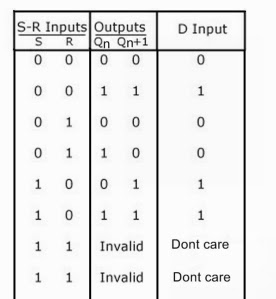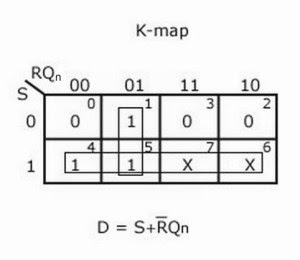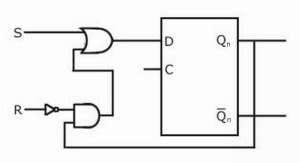### 2. Conversion of D flip-flop into JK flip-flop:

In case of conversion of D flip-flop to JK flip-flop, we have to use J and K as the external inputs and D as the input of the actual flip-flop. J, K, and Qn make eight possible combinations. Express D in terms of J, K, and Qn.

The conversion table, K-Maps and logic diagram for the conversion of D flip-flop into JK flip-flop is shown below: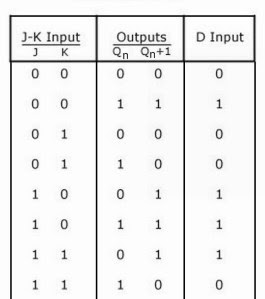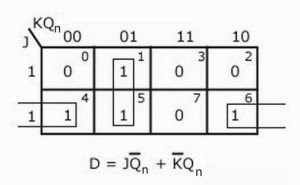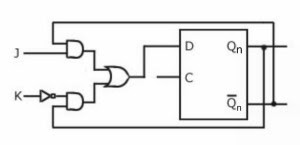You may also like: